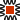#### Comparing "DB's The Easy Way" to Resistor Color Codes:

There is a interesting comparision that I have found between decoding resistor color codes and decoding Decibel values:

Resistor Color Codes:

• On resistors, the first two color bands describe a particular value, without a decimal point description

• i.e. the digits "39" can be 3.9 or 39 or 390

• the 3rd band is called "The Multiplier", and this is what actually determines the composite value and range of that resistor.

{on a side note, I taught fluent color-code in 18 minutes, in the classroom for over 30yrs}

Decibel Coding (or Decoding):

• The "Basic Decibel Value" is the Value that expresses or converts Logarithymically to a "Factor" for gain or loss.

• The number to the left of that Basic Decibel Value is "The Multiplier", as a power of the Base 10x.

With "1" simply being a Multiplier of 10 times the Factor,

or with "2" simply being a Multplier of 100 times the Factor,

or with "3" simply being a Multiplier of 1,000 times the Factor .

• Actually, the entire number expressed is actually a Logarithic Value as an exponent of the Base 10x,

but considering that there is a "hidden decimal point" involved.

i.e. 24Db would be considered as 102.4 (note the decimal point between the 2.4)How to determine Decibel Values and Examples: (DB_Tables pdf, or DB_Tables.html)
(no points discounted for speiling errers)

"Comparing.nnn" - ddf – 02/10/2021